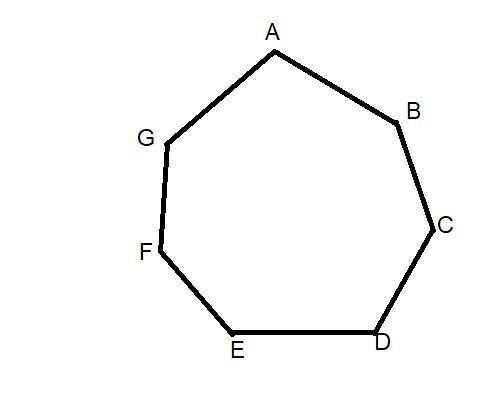Courses
Courses for Kids
Free study material
Free LIVE classes
MoreLIVE
Join Vedantu’s FREE Mastercalss

# How many sides and angles does a heptagon have?$A.{\text{ }}7 \\ B.{\text{ 11}} \\ {\text{C}}{\text{. 5}} \\ {\text{D}}{\text{. 12}} \\$Verified
363.6k+ views
Hint: Here in this question we go through the properties of polygon. We know many types of polygon. For this question we should have to recall the properties of heptagon to find the number of sides.

We have to find out the number of sides in the heptagon. For this we made the diagram of heptagon by recalling the definition of heptagon.
A heptagon is a polygon with 7 sides and 7 angles. Sometimes the heptagon is also known as “heptagon”. All the sides of a heptagon meet with each other end to end to form a shape.
By the help of diagrams we can also name the sides.
The sides are AB, BC, CD, DE, EF, FG, and GA.
By this definition we can say that, the number of heptagon sides = 7.
So option A is the correct answer.

Note: Whenever we face such a type of question. We have to go through the definition of that figure. And draw the diagram of that figure by the definition and then count the number of sides you will also find the number of sides in the definition.Last updated date: 28th Sep 2023
Total views: 363.6k
Views today: 7.63k